Download Presentation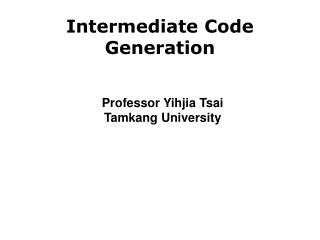Intermediate Code Generation

# Intermediate Code Generation - PowerPoint PPT Presentation

Intermediate Code Generation. Professor Yihjia Tsai Tamkang University. Introduction. Intermediate representation (IR) Generally a program for an abstract machine (can be assembly language or slightly above) Easy to produce and translate into target code Why?I am the owner, or an agent authorized to act on behalf of the owner, of the copyrighted work described.
Download Presentation## Intermediate Code Generation

An Image/Link below is provided (as is) to download presentation

Download Policy: Content on the Website is provided to you AS IS for your information and personal use and may not be sold / licensed / shared on other websites without getting consent from its author.While downloading, if for some reason you are not able to download a presentation, the publisher may have deleted the file from their server.

- - - - - - - - - - - - - - - - - - - - - - - - - - E N D - - - - - - - - - - - - - - - - - - - - - - - - - -
Presentation Transcript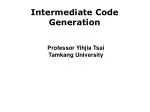### Intermediate Code Generation

Professor Yihjia Tsai

Tamkang University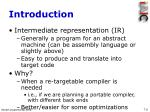Introduction
• Intermediate representation (IR)
• Generally a program for an abstract machine (can be assembly language or slightly above)
• Easy to produce and translate into target code
• Why?
• When a re-targetable compiler is needed
• i.e., if we are planning a portable compiler, with different back ends
• Better/easier for some optimizations
• Machine code can be more complex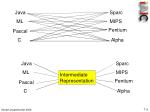Java

Sparc

ML

MIPS

Pentium

Pascal

C

Alpha

Java

Sparc

ML

MIPS

Intermediate

Representation

Pentium

Pascal

C

Alpha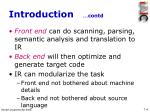Introduction …contd
• Front end can do scanning, parsing, semantic analysis and translation to IR
• Back end will then optimize and generate target code
• IR can modularize the task
• Front end not bothered about machine details
• Back end not bothered about source language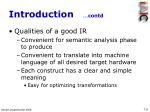Introduction …contd
• Qualities of a good IR
• Convenient for semantic analysis phase to produce
• Convenient to translate into machine language of all desired target hardware
• Each construct has a clear and simple meaning
• Easy for optimizing transformations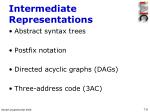Intermediate Representations
• Abstract syntax trees
• Postfix notation
• Directed acyclic graphs (DAGs)
• Three-address code (3AC)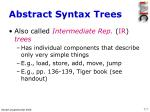Abstract Syntax Trees
• Also called Intermediate Rep. (IR) trees
• Has individual components that describe only very simple things
• E.g., load, store, add, move, jump
• E.g., pp. 136-139, Tiger book (see handout)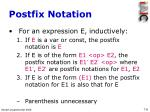Postfix Notation
• For an expression E, inductively:
• If E is a var or const, the postfix notation is E
• If E is of the form E1 <op> E2, the postfix notation is E1’ E2’ <op> where E1’, E2’ are postfix notations for E1, E2
• If E is of the form (E1) then the postfix notation for E1 is also that for E
• Parenthesis unnecessary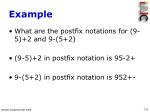Example
• What are the postfix notations for (9-5)+2 and 9-(5+2)
• (9-5)+2 in postfix notation is 95-2+
• 9-(5+2) in postfix notation is 952+-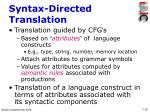Syntax-Directed Translation
• Translation guided by CFG’s
• Based on “attributes” of language constructs
• E.g., type, string, number, memory location
• Attach attributes to grammar symbols
• Values for attributes computed by semantic rules associated with productions
• Translation of a language construct in terms of attributes associated with its syntactic componentsSyntax-Directed Translation …contd
• Two notations for associating semantic rules with productions in a CFG
• Syntax-directed definitions
• High-level specs, details hidden, order of translation unspecified
• Translation schemes
• Order of translations specified, more details shown
• [Dragon book: Section 2.3 and Chapter 5]Syntax-Directed Definitions
• For each grammar symbol: associate a set of attributes (synthesized and inherited)
• For each production: a semantic rule defines the values of attribute at the parse-tree node used at that node
• Grammar + set of semantic rulesAnnotated Parse Tree
• A parse tree showing attribute value at each node
• Used for translation (which is an inputoutput mapping)
• For input x, construct parse tree for x
• If a node n in tree is labeled by symbol Y
• Value of attribute p of Y at node n denoted as Y.p
• Value of Y.p computed using semantic rule for attribute p associated with the Y-production at nSynthesized Attributes
• An attribute is synthesized if its value at a parse tree node is determined from those at the child nodes
• Can be evaluated with a single bottom-up tree traversal (e.g., depth-first traversal)
• A syntax-directed definition that uses these exclusively is said to be an s-attributed definitionExample 1

Translating expressions into postfix

“.t” is a string valued attribute, || is concatenationExample 1 …contd

expr.t = 95-2+

expr.t = 95-

term.t = 2

expr.t = 9

term.t = 5

term.t = 9

9

-

5

+

2

Annotated parse tree corresponding to “9-5+2”Example 2

Syntax-directed definition for desk calculator program

Draw the annotated parse tree for “3*5+4 \$”Example 2 …contd

L

\$

E.Val = 19

E.val = 15

+

T.val=4

T.val = 15

F.val=4

T.val=3

T.val=5

*

F.val=3

F.val=5

digit.lexval=4

digit.lexval=3

digit.lexval=5

Annotated parse tree corresponding to “3*5+4 \$”Inherited Attributes
• Value at a node is defined using attributes at siblings and/or parent of the node
• Useful for tracking the context of a construct
• E.g., decide whether address or value of a var is needed by keeping track of whether it appears on RHS or LHS of an assignmentExample

Syntax-directed definition with inherited attribute

L.in for declaration of variables of type int or real

Draw the annotated parse tree for “real id1, id2, id3”Example …contd

D

L.in = real

T.type = real

L.in = real

,

id3

real

L.in = real

,

id2

id1

Annotated parse tree for “real id1, id2, id3” with

inherited attribute in at each node LTranslation Schemes
• Semantic actions embedded within RHS of productions
• Unlike syntax-directed definitions, order of evaluation of semantic rules explicitly shown
• Action to be taken shown by enclosing in { }
• E.g., rterm term { print (‘+’) } rterm1
• In a parse tree in this context, an action is shown by an extra child node & dashed edgeDepth-First Order
• L-attributed definitions
• Attributes can be always evaluated in depth-first order (left-to-right)
• Translation schemes with restrictions motivated by L-attributed definitions ensure that an attribute value is available when an action refers to it
• E.g., when only synthesized attributes existExample
• Translation scheme that maps infix expressions with addition/subtraction into corresponding postfix expressions

E → T R

R → addop T { print(addop.lexeme) } R1 | Λ

R → subop T { print(subop.lexeme) } R2 | Λ

T → num{ print(num.val) }

• Show the parse tree for “9-5+2”Example …contd

E

R

T

-

T

{ print (‘-’) }

R

9

{ print (‘9’) }

+

T

{ print (‘+’) }

R

5

{ print (‘5’) }

Λ

2

{ print (‘2’) }

Parse tree for “9-5+2” showing actions; when performed in depth-first order, prints “95-2+”Emitting a Translation
• For simple syntax-directed definitions, implementation possible with translation schemes where actions print additional strings in the order of appearance
• [Simple: string representing the translation of the non-terminal on LHS of each production is the concatenation of translations of non-terminals on the RHS, in the same order as in the production]Example
• A translation scheme derived from Example in slide 7-15

expr → expr + term { print (‘+’) }

expr → expr – term { print (‘-’) }

expr → term

term → 0 { print (‘0’) }

term → 1 { print (‘1’) }

term → 9 { print (‘9’) }Example …contd

expr

+

{ print (‘+’) }

expr

term

-

{ print (‘-’) }

expr

term

2

{ print (‘2’) }

term

9

{ print (‘9’) }

5

{ print (‘5’) }

Actions translating “9-5+2” into “95-2+”Constructing Syntax Trees
• Syntax-directed definitions can be used
• Recall: syntax tree is a condensed form of parse tree
• Operators, keywords appear as interior nodes
• Construction: similar to postfix notation
• For a subexpression, create a node for each operator and operand
• Children of operator node represent operands (as subexpressions) of that operatorNodes in a Syntax Tree
• A node is like a record with many fields:
• label, pointers to operand nodes, value etc.,
• 3 basic functions to create nodes
• mknode(op, left, right): operator node with label op, two pointer fields left and right
• mkleaf(id, entry): ID node with label id and field entry pointing to symbol-table entry
• mkleaf(num, val): a NUM node with label num and value field containing value of numberExample
• From Example 5.7, p. 288
• What is the sequence of calls to create the syntax tree for the expression “a – 4 + c” ?

p1 = mkleaf(id, entry_a);

p2 = mkleaf(num, 4);

p3 = mknode(‘-’, p1, p2);

p4 = mkleaf(id, entry_c);

p5 = mknode(‘+’, p3, p4);

What is the syntax tree?Constructing Syntax Trees …contd
• A syntax-directed definition may be used for constructing a syntax tree
• Semantic rules: calls to functions mknode( ) and mkleaf( )
• E.g., for the production, E  E1 + T, we may have the semantic rule

E.nptr = mknode(‘+’, E1.nptr, T.nptr)

• Example 5.8, p. 289DAGs for Expressions
• A dag for an expression identifies common subexpressions
• Unlike a syntax tree, a node for a common subexpression may have > 1 parent node
• E.g., “a + a * (b-c) + (b-c) * d”
• Fig. 5.11, p.291
• How to create a dag, given an expression?
• Check if an identical node already exists
• Example 5.9, p. 291Review
• Example: for the assignment statement, a = b * -c + b * -c,

give a syntax tree, dag and postfix notation

• Fig. 8.2, p. 464• 3AC is a sequence of statements of the general form

x := y <op> z

• x, y, z are names, const’s, generated temp’s
• <op> is any operator (arithmetic, logical)
• 3AC means each statement usually has 3 addresses (2 for operands, 1 for the result)Examples
• Given the expression, x+y*z the 3AC

t1 := y * z t2 := x + t1

• Show 3AC for (a) syntax tree, (b) dag discussed earlier in slide 7-34 (Fig. 8.2)
• Fig. 8.5, p. 4663AC …contd
• A name in a program replaced by a pointer to a symbol table entry for that name
• 3AC statements are like assembly code
• There are flow-control statements
• They can have symbolic labels
• A label represents the index of a 3AC statement in an array containing the intermediate codeTypes of 3AC Statements
• Assignment statements with binary operators (arithmetic or logical)
• Of the form x:= y <op> z
• Assignment statements with unary operators (minus, logical not, shift etc.,)
• Of the form x:= <op> y
• Copy statements
• Of the form x := yTypes of 3AC Statements …contd
• Unconditional jump: goto L
• Statement with label L to be executed next
• Conditional jump: if x <relop> y goto L
• A relational operator (<, =, >= …) is applied to x and y
• If the relation holds, statement with label L executed next
• If not, statement following it is executedTypes of 3AC Statements …contd
• Function calls: param x ,call p, n and return y
• “returny” is optional
• E.g., for call p(x1, x2, …, xn) the 3AC will be

param x1

param x2

param xn

call p, nTypes of 3AC Statements …contd
• Indexed assignments: x := y[i] , x[i] := y
• In x:=y[i] : x is set to the value in location i units beyond memory location y
• In x[i]:=y : value in location i units beyond memory location x is set to the value of y
• x, y and i are data objectsTypes of 3AC Statements …contd
• Address & pointer assignments: x := &y , x := *y , *x := y
• In x:= &y : x is set to be the location of y
• y denotes an l-value, x is a pointer name
• In x:= *y : (r-value of) x is set to the value in location pointed by y
• y is a pointer; r-value of y is a location
• In *x:= y : (r-value of) object pointed by x is set to (the r-value of) ySyntax-Dir. Translation into 3AC
• When 3AC code is generated, temp names are made up for interior nodes in syntax tree
• E.g., for E  E1 + E2, value of E on LHS will be computed to a new temp t
• Example
• Fig. 8.6, Fig 8.7 on p. 469Implementation of 3AC
• 3AC is an abstract form
• Can be implemented in a compiler as records
• (with fields for operator and operands)
• Three representations
• Triples
• Indirect triples• A record structure with 4 fields
• op, arg1, arg2 and result
• Examples
• For x := y op z we have:
• y in arg1, z in arg2 and x in result
• For unary operators, arg2 not used
• For param operator, arg2 and result unused
• Fig. 8.8(a), p. 471 for a:= b* -c + b* -c
• Content of fields are pointers to ST entries(b) Triples
• Temps generated in quadruples must be entered in symbol table
• To avoid this, we can refer to a temp value by the location of the relevant statement
• We can have records with only 3 fields
• op, arg1 and arg2
• Fields arg1 and arg2 can be pointers to ST entries or to triple structure for temp values
• Example: Fig 8.8(b), Fig. 8.9 on p. 471(c) Indirect Triples
• Listing of pointers to triples, rather than triples themselves
• Example
• We can use an array to list pointers to triples in the desired order
• Example: Fig 8.10 on p. 472Translating Language Constructs
• Balance of Chapter 8 in Dragon book covers details on implementing:
• Declarations, scope
• Assignments, array elements, fields in records
• Boolean expressions
• Case statements
• Label renaming (called backpatching)
• Function calls